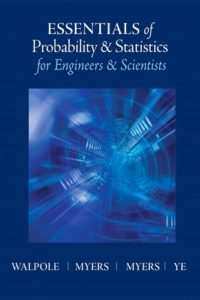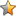اسم المؤلف
Ronald E. Walpole, Raymond H. Myers, Sharon L. Myers, Keying Ye
التاريخ
25 سبتمبر 2016
المشاهدات
52
التقييمLoading...

Probability and Statistics for Engineers and Scientists
Ronald E Walpole
Roanoke College
Raymond H Myers
Virginia Tech
Sharon L Myers
Keying Ye
University of Texas at San Antonio
Contents
Preface xv
1 Introduction to Statistics and Data Analysis 1
1.1 Overview: Statistical Inference, Samples, Populations, and the
Role of Probability 1
1.2 Sampling Procedures; Collection of Data 7
1.3 Measures of Location: The Sample Mean and Median 11
Exercises 13
1.4 Measures of Variability 14
Exercises 17
1.5 Discrete and Continuous Data 17
1.6 Statistical Modeling, Scientific Inspection, and Graphical Diagnostics 18
1.7 General Types of Statistical Studies: Designed Experiment,
Observational Study, and Retrospective Study 27
Exercises 30
2 Probability 35
2.1 Sample Space 35
2.2 Events 38
Exercises 42
2.3 Counting Sample Points 44
Exercises 51
2.4 Probability of an Event 52
Exercises 59
2.6 Conditional Probability, Independence, and the Product Rule 62
Exercises 69
2.7 Bayes’ Rule 72
Exercises 76
Review Exercises 77viii Contents
2.8 Potential Misconceptions and Hazards; Relationship to Material
in Other Chapters 79
3 Random Variables and Probability Distributions 81
3.1 Concept of a Random Variable 81
3.2 Discrete Probability Distributions 84
3.3 Continuous Probability Distributions 87
Exercises 91
3.4 Joint Probability Distributions 94
Exercises 104
Review Exercises 107
3.5 Potential Misconceptions and Hazards; Relationship to Material
in Other Chapters 109
4 Mathematical Expectation 111
4.1 Mean of a Random Variable 111
Exercises 117
4.2 Variance and Covariance of Random Variables 119
Exercises 127
4.3 Means and Variances of Linear Combinations of Random Variables 128
4.4 Chebyshev’s Theorem 135
Exercises 137
Review Exercises 139
4.5 Potential Misconceptions and Hazards; Relationship to Material
in Other Chapters 142
5 Some Discrete Probability Distributions 143
5.1 Introduction and Motivation 143
5.2 Binomial and Multinomial Distributions 143
Exercises 150
5.3 Hypergeometric Distribution 152
Exercises 157
5.4 Negative Binomial and Geometric Distributions 158
5.5 Poisson Distribution and the Poisson Process 161
Exercises 164
Review Exercises 166
5.6 Potential Misconceptions and Hazards; Relationship to Material
in Other Chapters 169Contents ix
6 Some Continuous Probability Distributions 171
6.1 Continuous Uniform Distribution 171
6.2 Normal Distribution 172
6.3 Areas under the Normal Curve 176
6.4 Applications of the Normal Distribution 182
Exercises 185
6.5 Normal Approximation to the Binomial 187
Exercises 193
6.6 Gamma and Exponential Distributions 194
6.7 Chi-Squared Distribution 200
6.8 Beta Distribution 201
6.9 Lognormal Distribution 201
6.10 Weibull Distribution (Optional) 203
Exercises 206
Review Exercises 207
6.11 Potential Misconceptions and Hazards; Relationship to Material
in Other Chapters 209
7 Functions of Random Variables (Optional) 211
7.1 Introduction 211
7.2 Transformations of Variables 211
7.3 Moments and Moment-Generating Functions 218
Exercises 222
8 Fundamental Sampling Distributions and
Data Descriptions 225
8.1 Random Sampling 225
8.2 Some Important Statistics 227
Exercises 230
8.3 Sampling Distributions 232
8.4 Sampling Distribution of Means and the Central Limit Theorem 233
Exercises 241
8.5 Sampling Distribution of S2 243
8.6 t-Distribution 246
8.7 F -Distribution 251
8.8 Quantile and Probability Plots 254
Exercises 259
Review Exercises 260
8.9 Potential Misconceptions and Hazards; Relationship to Material
in Other Chapters 262x Contents
9 One- and Two-Sample Estimation Problems 265
9.1 Introduction 265
9.2 Statistical Inference 265
9.3 Classical Methods of Estimation 266
9.4 Single Sample: Estimating the Mean 269
9.5 Standard Error of a Point Estimate 276
9.6 Prediction Intervals 277
9.7 Tolerance Limits 280
Exercises 282
9.8 Two Samples: Estimating the Difference between Two Means 285
9.9 Paired Observations 291
Exercises 294
9.10 Single Sample: Estimating a Proportion 296
9.11 Two Samples: Estimating the Difference between Two Proportions 300
Exercises 302
9.12 Single Sample: Estimating the Variance 303
9.13 Two Samples: Estimating the Ratio of Two Variances 305
Exercises 307
9.14 Maximum Likelihood Estimation (Optional) 307
Exercises 312
Review Exercises 313
9.15 Potential Misconceptions and Hazards; Relationship to Material
in Other Chapters 316
10 One- and Two-Sample Tests of Hypotheses 319
10.1 Statistical Hypotheses: General Concepts 319
10.2 Testing a Statistical Hypothesis 321
10.3 The Use of P -Values for Decision Making in Testing Hypotheses 331
Exercises 334
10.4 Single Sample: Tests Concerning a Single Mean 336
10.5 Two Samples: Tests on Two Means 342
10.6 Choice of Sample Size for Testing Means 349
10.7 Graphical Methods for Comparing Means 354
Exercises 356
10.8 One Sample: Test on a Single Proportion 360
10.9 Two Samples: Tests on Two Proportions 363
Exercises 365
10.10 One- and Two-Sample Tests Concerning Variances 366
Exercises 369
10.11 Goodness-of-Fit Test 370
10.12 Test for Independence (Categorical Data) 373Contents xi
10.13 Test for Homogeneity 376
10.14 Two-Sample Case Study 379
Exercises 382
Review Exercises 384
10.15 Potential Misconceptions and Hazards; Relationship to Material
in Other Chapters 386
11 Simple Linear Regression and Correlation 389
11.1 Introduction to Linear Regression 389
11.2 The Simple Linear Regression Model 390
11.3 Least Squares and the Fitted Model 394
Exercises 398
11.4 Properties of the Least Squares Estimators 400
11.5 Inferences Concerning the Regression Coefficients 403
11.6 Prediction 408
Exercises 411
11.7 Choice of a Regression Model 414
11.8 Analysis-of-Variance Approach 414
11.9 Test for Linearity of Regression: Data with Repeated Observations 416
Exercises 421
11.10 Data Plots and Transformations 424
11.11 Simple Linear Regression Case Study 428
11.12 Correlation 430
Exercises 435
Review Exercises 436
11.13 Potential Misconceptions and Hazards; Relationship to Material
in Other Chapters 442
12 Multiple Linear Regression and Certain
Nonlinear Regression Models 443
12.1 Introduction 443
12.2 Estimating the Coefficients 444
12.3 Linear Regression Model Using Matrices 447
Exercises 450
12.4 Properties of the Least Squares Estimators 453
12.5 Inferences in Multiple Linear Regression 455
Exercises 461
12.6 Choice of a Fitted Model through Hypothesis Testing 462
12.7 Special Case of Orthogonality (Optional) 467
Exercises 471
12.8 Categorical or Indicator Variables 472xii Contents
Exercises 476
12.9 Sequential Methods for Model Selection 476
12.10 Study of Residuals and Violation of Assumptions (Model Checking) 482
12.11 Cross Validation, Cp, and Other Criteria for Model Selection 487
Exercises 494
12.12 Special Nonlinear Models for Nonideal Conditions 496
Exercises 500
Review Exercises 501
12.13 Potential Misconceptions and Hazards; Relationship to Material
in Other Chapters 506
13 One-Factor Experiments: General 507
13.1 Analysis-of-Variance Technique 507
13.2 The Strategy of Experimental Design 508
13.3 One-Way Analysis of Variance: Completely Randomized Design
(One-Way ANOVA) 509
13.4 Tests for the Equality of Several Variances 516
Exercises 518
13.5 Single-Degree-of-Freedom Comparisons 520
13.6 Multiple Comparisons 523
Exercises 529
13.7 Comparing a Set of Treatments in Blocks 532
13.8 Randomized Complete Block Designs 533
13.9 Graphical Methods and Model Checking 540
13.10 Data Transformations in Analysis of Variance 543
Exercises 545
13.11 Random Effects Models 547
13.12 Case Study 551
Exercises 553
Review Exercises 555
13.13 Potential Misconceptions and Hazards; Relationship to Material
in Other Chapters 559
14 Factorial Experiments (Two or More Factors) 561
14.1 Introduction 561
14.2 Interaction in the Two-Factor Experiment 562
14.3 Two-Factor Analysis of Variance 565
Exercises 575
14.4 Three-Factor Experiments 579
Exercises 586Contents xiii
14.5 Factorial Experiments for Random Effects and Mixed Models 588
Exercises 592
Review Exercises 594
14.6 Potential Misconceptions and Hazards; Relationship to Material
in Other Chapters 596
15 2k Factorial Experiments and Fractions 597
15.1 Introduction 597
15.2 The 2k Factorial: Calculation of Effects and Analysis of Variance 598
15.3 Nonreplicated 2k Factorial Experiment 604
Exercises 609
15.4 Factorial Experiments in a Regression Setting 612
15.5 The Orthogonal Design 617
Exercises 625
15.6 Fractional Factorial Experiments 626
15.7 Analysis of Fractional Factorial Experiments 632
Exercises 634
15.8 Higher Fractions and Screening Designs 636
15.9 Construction of Resolution III and IV Designs with 8, 16, and 32
Design Points 637
15.10 Other Two-Level Resolution III Designs; The Plackett-Burman
Designs 638
15.11 Introduction to Response Surface Methodology 639
15.12 Robust Parameter Design 643
Exercises 652
Review Exercises 653
15.13 Potential Misconceptions and Hazards; Relationship to Material
in Other Chapters 654
16 Nonparametric Statistics 655
16.1 Nonparametric Tests 655
16.2 Signed-Rank Test 660
Exercises 663
16.3 Wilcoxon Rank-Sum Test 665
16.4 Kruskal-Wallis Test 668
Exercises 670
16.5 Runs Test 671
16.6 Tolerance Limits 674
16.7 Rank Correlation Coefficient 674
Exercises 677
Review Exercises 679xiv Contents
17 Statistical Quality Control 681
17.1 Introduction 681
17.2 Nature of the Control Limits 683
17.3 Purposes of the Control Chart 683
17.4 Control Charts for Variables 684
17.5 Control Charts for Attributes 697
17.6 Cusum Control Charts 705
Review Exercises 706
18 Bayesian Statistics 709
18.1 Bayesian Concepts 709
18.2 Bayesian Inferences 710
18.3 Bayes Estimates Using Decision Theory Framework 717
Exercises 718
Bibliography 721
Appendix A: Statistical Tables and Proofs 725
Appendix B: Answers to Odd-Numbered Non-Review
Exercises 769
Index
كلمة سر فك الضغط : books-world.net